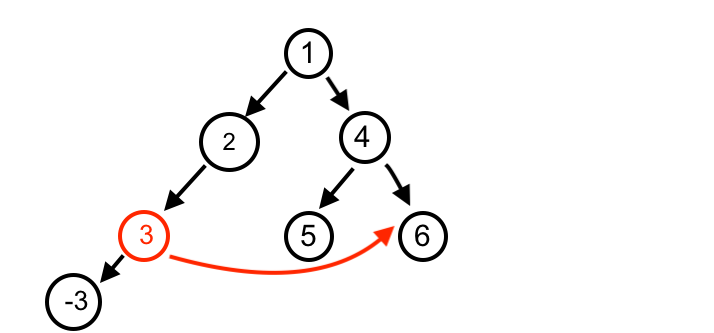# Errata for Data Structures & Algorithms in Swift, 4th Edition

Creating this topic to catch any typos and bugs in the 4th Edition of Data Structures & Algorithms in Swift.

1 Like

How do I fix this error? Why doesn’t example work for this and the Previous chapter?:

error: Node.playground:24:1: error: cannot find ‘example’ in scope

example(of: “creating and linking nodes”) {

^~~~~~~

Regarding the Performance Analysis section for removing elements of a LinkedList… Shouldn’t removeLast() be O(n) and not O(1)? Since the implementation of removeLast() involves iterating over every node in the list starting at the head?

1 Like

Chapter 36: Graph

Why AdjancencyList and Matrix is a class and not struct in the book?

public class AdjacencyList<T: Hashable>: Graph {
private var adjacencies: [Vertex: [Edge]] = [:]
public init() {}
// more to come …
}

What are the advantage of this? and what is wrong if we make it struct?

Yes, that’s correct. The most recent version of the book states that:

`removeLast` requires you to traverse all the way down the list. This makes for an O(n) operation, which is relatively expensive.

1 Like

Chapter 22, page 229 (Pdf version), removing the root node from the heap. “A remove operation will remove the maximum value at the root node. To do so, you must first swap the root node with the last element in the heap.” : In the diagram on page 228, the maximum value is “10” and the last node is “3”, on page 229 the lefthand diagram at the top of the page has swapped “10” with “7”. There was no “7”. In the immediate diagram to the right at the top of the page, the “7” has turned into a “3”.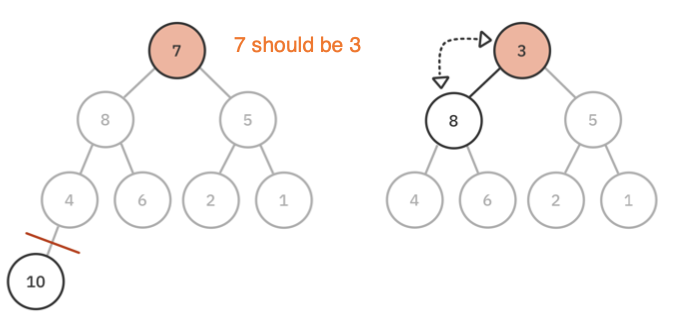1 Like

Chapter 36: Graphs. Page 338 and 339 (PDF version). Error in the adjacency list diagram on page 339. For the “Tokyo” vertex, the adjacency list has two “Detroit”, missing “Kong Kong” in place of a “Detroit”.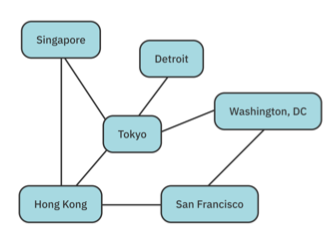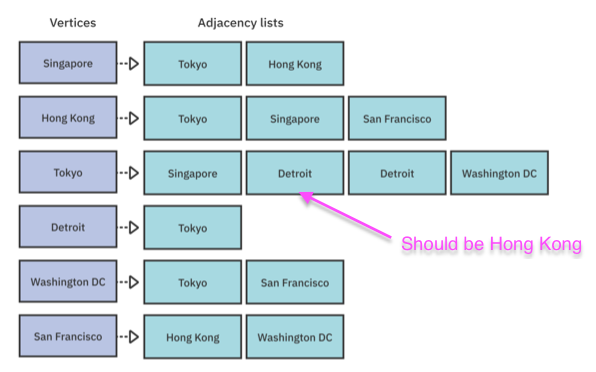Thank you for taking time to report these! We will get them updated for the next edition!

I think I found a contradiction in the Binary Search Tree definitions in Chapter 14 and Chapter 15.

Chapter 14 Binary Search Tree says:

• The value of a left child must be less than the value of its parent.
• Consequently, the value of a right child must be greater than or equal to the value of its parent.

But Chapter 15 Binary Search Tree Challenges in “Solution to Challenge 1” says:

A binary search tree is a tree where every left child is less than or equal to its parent, and every right child is greater than its parent.

So chapter 14 says that values equal to the parent should be on the right side but chapter 15 says that they should be on the left side. I think the issue is that there is no universally agreed upon definition of a BST. Some implementations allow duplicates and some do not. When they do allow duplicates, there’s no agreement as to what branch a duplicate should go under. But it would still be helpful for the book to have one consistent definition on this matter.

Are you kidding me that no one has answered this question? The examples do NOT WORK directly from source and this basic question is being ignored? Really?

Hi! I guess the table below, summarizing complexity is still wrong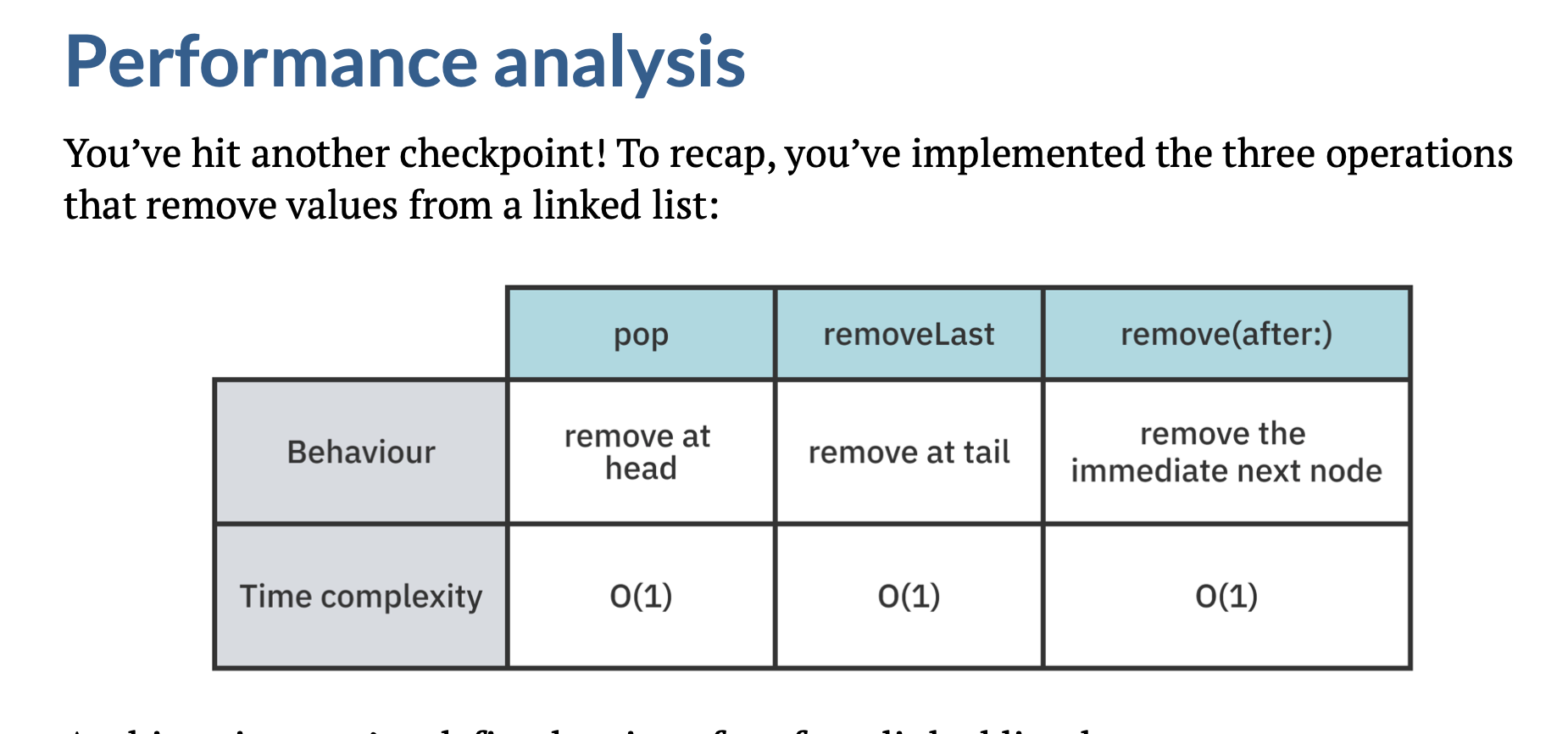Page 67 - Chapter 6 - LInkedList - v4.0

`removeLast` should be O(n) and not O(1).

1 Like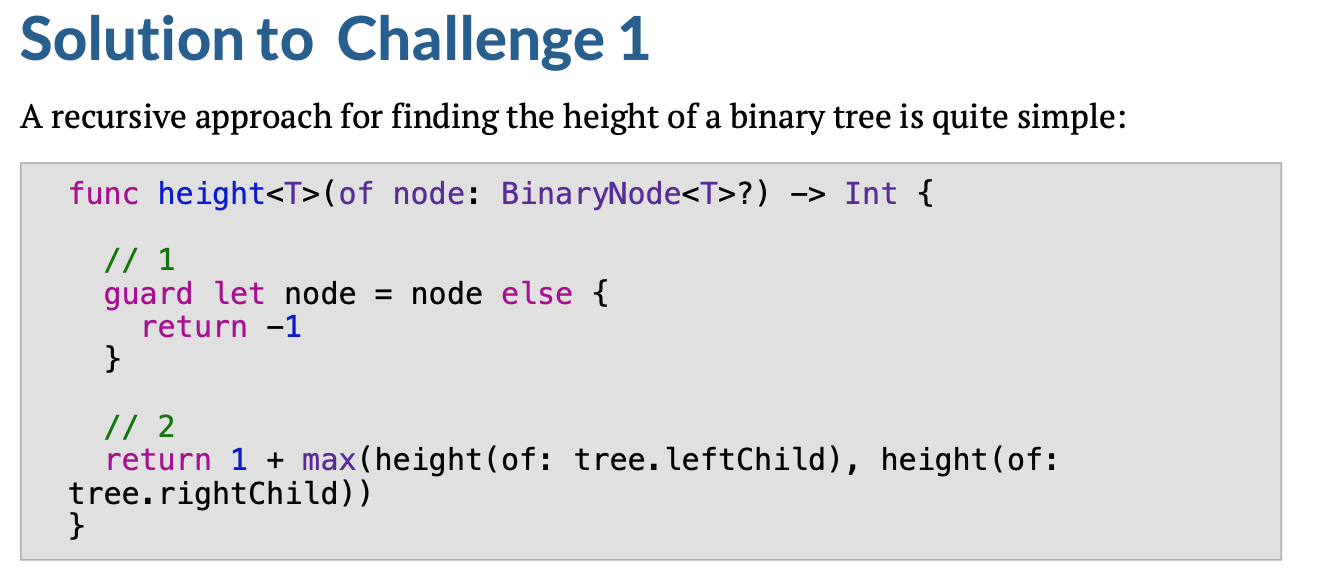This function is wrong, because it iterate recursively on `tree` (the global variable) and not on `node` (the input one).

The right function should be

``````func height<T>(of node: BinaryNode<T>?) -> Int {
// 1
guard node != nil else {
return -1
}
// 2
return 1 + max(height(of: node?.leftChild), height(of:node?.rightChild))
}
``````

Chapter 13 - page 150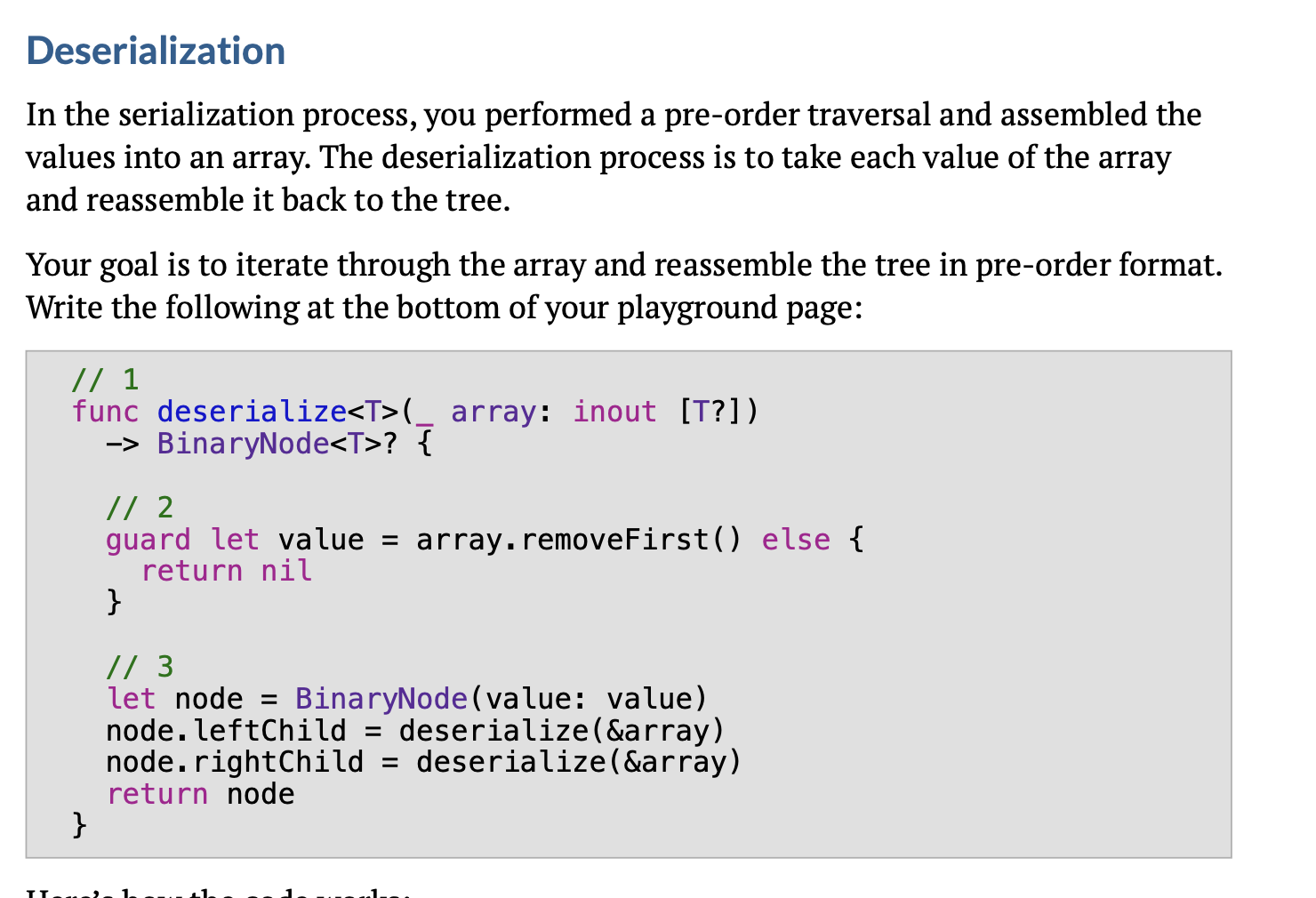Chapter 13 - page 152 is missing the empty check for the array in `deserialize`

Chapter 21, Binary Search Challenges
Challenge #2 Solution shows “An unoptimized but elegant solution” that “is quite simple”.
It is however wrong.

The solution returns the range
`firstIndex(of:)..<lastIndex(of:)`
while in fact it should return the range
`firstIndex(of:)..<(lastIndex(of:) + 1)`
so that the last index is also included in the half-open interval

In the LinkedList chapter, under the section for removing values, it says there are three operations: 1. `pop`
`removeLast`
`remove(at:)`

In the actual body of the text, the operation discussed is `remove(after:)`1 Like

The adjacency list and matrix are nicely explained in kuickcode dot com
List<int[]>[] graph = new List[n];
for (int i = 0; i < n; i++) {
graph[i] = new ArrayList<>();
}
for (int i = 0; i < edges.length; i++) {
int[] edge = edges[i];
int u = edge;
int v = edge;
int w = distance[i];
//If there is an undirected edge from vertex u to vertex v, then we need install v as child of u and vice versa.